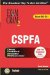# Chapter 18. Answer Key 2

1. A, E

2. B, C

3. C

4. C

5. C

6. B

7. A, C, D

8. A

9. A, E

10. C

11. A, C, D

12. A

13. A, B

14. D, E

15. C

16. A

17. B

18. D

19. B

20. C

21. A, B, D

22. B

23. D

24. B

25. C

26. A, B, D, E

27. E

28. C

29. A, D

30. A, B, D

31. C

32. B

33. A, B, D, E

34. E

35. A, B

36. B, D

37. B, C, E

38. D

39. B, C, D

40. A, B, C, E

41. A, C, E

42. A

43. D

44. B, C, D

45. D

46. D

47. A, B, E

48. A, B

49. C, E

50. A, D

51. A

52. B

53. A, B, D, E

54. B, D, E

55. A, C, D

56. D

57. B, C, D

58. B, C, D

59. A, B, D, E

60. B

61. A

62. C

63. C

64. C

65. A

66. D

67. B, C

68. C

69. A, B

70. D

71. A, B, C

72. B

73. C

74. A

75. A, C, E